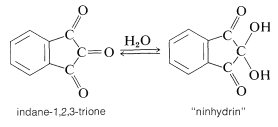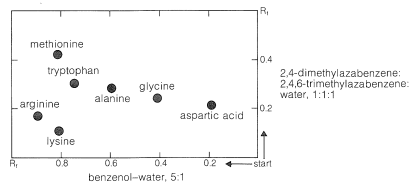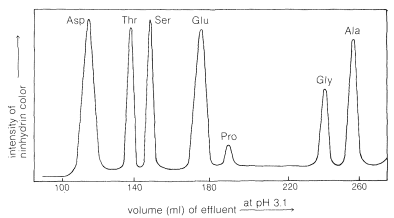# 25.4: Analysis of Amino Acids

$$\newcommand{\vecs}{\overset { \rightharpoonup} {\mathbf{#1}} }$$ $$\newcommand{\vecd}{\overset{-\!-\!\rightharpoonup}{\vphantom{a}\smash {#1}}}$$$$\newcommand{\id}{\mathrm{id}}$$ $$\newcommand{\Span}{\mathrm{span}}$$ $$\newcommand{\kernel}{\mathrm{null}\,}$$ $$\newcommand{\range}{\mathrm{range}\,}$$ $$\newcommand{\RealPart}{\mathrm{Re}}$$ $$\newcommand{\ImaginaryPart}{\mathrm{Im}}$$ $$\newcommand{\Argument}{\mathrm{Arg}}$$ $$\newcommand{\norm}{\| #1 \|}$$ $$\newcommand{\inner}{\langle #1, #2 \rangle}$$ $$\newcommand{\Span}{\mathrm{span}}$$ $$\newcommand{\id}{\mathrm{id}}$$ $$\newcommand{\Span}{\mathrm{span}}$$ $$\newcommand{\kernel}{\mathrm{null}\,}$$ $$\newcommand{\range}{\mathrm{range}\,}$$ $$\newcommand{\RealPart}{\mathrm{Re}}$$ $$\newcommand{\ImaginaryPart}{\mathrm{Im}}$$ $$\newcommand{\Argument}{\mathrm{Arg}}$$ $$\newcommand{\norm}{\| #1 \|}$$ $$\newcommand{\inner}{\langle #1, #2 \rangle}$$ $$\newcommand{\Span}{\mathrm{span}}$$$$\newcommand{\AA}{\unicode[.8,0]{x212B}}$$

## The Ninhydrin and Related Tests

In many kinds of research it is important to have simple and sensitive means for analysis of amino acids, particularly in small quantities. Detection of amino acids can be achieved readily by the "ninhydrin color test", whereby an alcoholic solution of the triketone, "ninhydrin", is heated with an amino acid and produces an intense blue-violet color. The sensitivity and reliability of this test is such that 0.1 micromole of amino acid gives a color intensity reproducible to a few per cent, provided that a reducing agent such as stannous chloride is present to prevent oxidation of the colored salt by dissolved oxygen.The color-forming reaction is interesting because most $$\alpha$$-amino acids give the same color irrespective of their structure.$$^4$$ The sequence of steps that leads to the color is as follows:A new, very sensitive method of detection and analysis of amino acids, which is useful down to the $$10^{-12}$$ mole (picomole) level, depends on the formation from $$\ce{RNH_2}$$ and "fluorescamine", $$1$$, of substances that are intensely fluorescent in ultraviolet light:## Paper Chromatography

Ninhydrin (or fluorescamine) is very useful in chromatographic methods for the analysis of amino acids. One of these is paper chromatography, wherein amino acids are separated as the consequence of differences in their partition coefficients between water and an organic solvent. The aqueous phase is held stationary in the pores of the paper because of strong interaction of the water with the hydroxyl functions of the cellulose. The differences in partition coefficients show up as differences in rates of migration on the surface of moist (but not wet) paper over which there is a slow flow of a water-saturated organic solvent. We shall discuss one of several useful modes of operation. In this example, a drop of the solution to be analyzed is placed on the corner of a sheet of moist paper (often filter paper), which is then placed in an apparatus like that of Figure 25-2, arranged so that the organic solvent can migrate upward by capillarity across the paper, carrying the amino acids with it along one edge. The acids that have the greatest solubility in the organic solvent move most rapidly and when the solvent reaches the top of the paper, the paper is removed, dried, and then turned sidewise, and a different solvent allowed to migrate upward. This double migration process gives a better separation of the amino acids than a single migration and results in concentration of the different amino acids in rather well-defined spots. These spots can be made visible by first drying and then spraying the paper with ninhydrin solution. The final result is as shown in Figure 25-3 and usually is quite reproducible under a given set of conditions. The identities of the amino acids that produce the various spots are established by comparison with the behavior of known mixtures.Figure 25-2: Diagram of apparatus used to develop a paper chromatogram. Paper is suspended from its top edge within an airtight container, here a glass box closed with a glass plate, having an atmosphere saturated with solvent vapor, the lower edge of paper dips into a trough containing the liquid solvent.Figure 25-3: Idealized two-dimensional paper chromatogram of a mixture of amino acids. The horizontal and vertical scales represent the distance of travel of a component of the mixture in a given solvent relative to that of the solvent itself. This is known as the $$R_f$$ value and is fairly constant for a particular compound in a given solvent. A rough identification of the amino acids present in the mixture may therefore be made on the bases of their $$R_f$$ values:Analysis by thin-layer chromatography (see Section 9-2B) can be carried out in the same way as paper chromatography. The partitioning is now between a solid stationary phase (the coating on the plate) and the moving solvent front.

## Ion-Exchange Chromatography

The advent of ion-exchange chromatography has revolutionized the separation and analysis of amino acids as well as that of many inorganic substances. As the name implies, it involves the exchange of ions between a stationary and a moving phase. The stationary phase is an insoluble polymer (or resin) having chains on which are located ionic functions such as sulfonate groups, $$\ce{-SO_3^-}$$ or quaternary ammonium groups, $$\ce{-} \overset{\oplus}{\ce{N}} \ce{R_3}$$. The counterions to these groups, such as $$\ce{Na}^\oplus$$ or $$\ce{Cl}^\ominus$$, are not bound to the resin and can be exchanged for other ions in the mobile phase as the mobile phase travels through the resin. A common application of this principle is in household water softeners, in which the calcium and magnesium ions in ordinary "hard" water are replaced by sodium ions from the resin (Equation 25-3). The resulting "soft" water can be freed of metal ions, if desired, by exchanging the $$\ce{Na}^\oplus$$ ions for protons (Equation 25-4):

$2 \left( \text{resin} \ce{-SO_3^-} \ce{Na^+} \right)+ \ce{Ca^{2+}} \rightleftharpoons 2 \left( \text{resin} \ce{-SO_3^-} \right) \ce{Ca^{2+}} + 2 \ce{Na^+} \tag{25-3}$

$\text{resin} \ce{-SO_3^-} \ce{H^+} \rightleftharpoons \text{resin} \ce{-SO_3^-} \ce{Na^+} + \ce{H^+} \tag{25-4}$

In strongly acidic solutions (pH $$\sim 0$$), the amine and carboxyl groups of an amino acid are completely protonated. This cationic form of the amino acid can be exchanged with the cations associated with the sulfonate groups of the resin:

$\text{resin} \ce{-SO_3^-} \ce{Na^+} + \ce{H_3} \overset{+}{\ce{N}} \ce{CRHCO_2H} \rightleftharpoons \text{resin} \ce{-SO_3^-} \ce{H_3} \overset{+}{\text{N}} \ce{CRHCO_2H} + \ce{Na^+} \tag{25-5}$

The process is reversible, and the amino acid cations can in turn be exchanged off the columns. However, different amino acids have different affinities for the resin, and these are considerably influenced by the pH of the moving phase (eluent). The basic amino acids (arginine, lysine), which form cations most readily, are more strongly held by cation-exchange resins than are acidic amino acids (aspartic and glutamic acids). There is a spectrum of affinities of the other amino acid cations for the resin between these extremes. Thus a mixture of amino acids can be separated by ion-exchange chromatography by elution with buffered aqueous solutions. The effluent from the column is mixed with ninhydrin solution and the intensity of the blue color is measured and plotted as a function of time at constant flow rates (Figure 25-4). The identity of an amino acid is determined by the volume of solvent required to elute the amino acid from the column, and the concentration is determined from the intensity of the color developed.Figure 25-4: Part of amino-acid chromatogram obtained by the method of automatic amino-acid analysis from a hydrolyzed sample of the enzyme ribonuclease. The component amino acids listed are present in the ratio Asp:Thr:Ser:Glu:Pro:Gly:Ala $$=$$ 15:10:15:12:4:3:12, as determined by peak intensity. The volume of effluent is a measure of the retention time of the amino acids on the column.

$$^4$$Proline and hydroxyproline are exceptions because neither has the necessary primary $$\ce{NH_2}$$ group needed for the reaction. However, these compounds do react with ninhydrin to give yellow compounds, and these colors can be used to identify them satisfactorily.